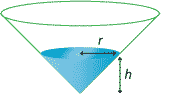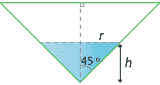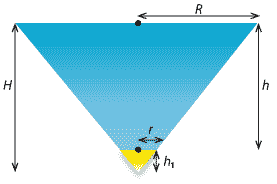## Content

### Related rates of change

Related rates of change are simply an application of the chain rule. In related-rate problems, you find the rate at which some quantity is changing by relating it to other quantities for which the rate of change is known.

In the following examples, we repeatedly use the result $\dfrac{dx}{dy} = \dfrac{1}{\dfrac{dy}{dx}},$ which is established and discussed in the module Introduction to differential calculus.

#### Example

An upturned cone with semivertical angle $$45^\circ$$ is being filled with water at a constant rate of 30 cm$$^3$$ per second.When the depth of the water is 60 cm, find the rate at which
1. $$h$$, the depth of the water, is increasing
2. $$r$$, the radius of the surface of the water, is increasing
3. $$S$$, the area of the water surface, is increasing.

#### SolutionThe volume $$V$$ of the water in the cone is given by

$V = \dfrac{1}{3}\pi r^2h.$

The cross-section of the cone is a right-angled isosceles triangle, and therefore $$r = h$$. Hence,

$V = \dfrac{1}{3}\pi r^3 = \dfrac{1}{3}\pi h^3.$
1. We seek $$\dfrac{dh}{dt}$$. Now $$V = \dfrac{1}{3}\pi h^3$$, and therefore $$\dfrac{dV}{dh} =\pi h^2$$. Hence, \begin{align*} \dfrac{dh}{dt} &= \dfrac{dh}{dV} \times \dfrac{dV}{dt} \\ &= \dfrac{1}{\pi h^2} \times 30 \\ &= \dfrac{30}{\pi h^2}. \end{align*}

When $$h = 60$$, we have $$\dfrac{dh}{dt} = \dfrac{1}{120\pi}$$ cm/s.

2. We have seen that $$r(t)=h(t)$$. Thus, when $$h = 60$$, we have $$\dfrac{dr}{dt} = \dfrac{1}{120\pi}$$ cm/s.
3. We seek $$\dfrac{dS}{dt}$$. The area of the water's surface is $S = \pi r^2.$ Therefore \begin{align*} \dfrac{dS}{dt} &= \dfrac{dS}{dr} \times \dfrac{dr}{dt} \\ &= 2\pi r \times \dfrac{30}{\pi r^2} \\ &= \dfrac{60}{r}. \end{align*}

When $$h = 60$$, we have $$r = 60$$, and so $$\dfrac{dS}{dt} = 1$$ cm$$^2$$/s.

#### Example

Variables $$x$$ and $$y$$ are related by the equation $$y = 2 - \dfrac{4}{x}$$. Given that $$x$$ and $$y$$ are functions of $$t$$ and that $$\dfrac{dx}{dt} = 10$$, find $$\dfrac{dy}{dt}$$ in terms of $$x$$.

#### Solution

If $$y = 2 - \dfrac{4}{x}$$, then $$\dfrac{dy}{dx} =\dfrac{4}{x^2}$$. Therefore \begin{align*} \dfrac{dy}{dt} &= \dfrac{dy}{dx}\times \dfrac{dx}{dt} \\ &= \dfrac{4}{x^2} \times 10 \\ &= \dfrac{40}{x^2}. \end{align*}

Related rates questions arise in many situations. The following demanding example involving the flow of liquids relates the rate of change of depth to the rate of change of volume.

#### Example

A vessel of water is in the form of a frustum of a cone with semivertical angle $$45^\circ$$. The bottom circle of the vessel is a hole of radius $$r$$ cm. Water flows from this hole at a velocity of $$c\sqrt{2gh}$$ cm/s, where $$c$$ is a constant and $$h$$ cm is the height of the surface of the water above the hole.

1. Find the rate in cm$$^3$$/s at which water flows from the vessel.
2. Find the rate in cm/s at which $$h$$ is increasing.#### Solution

We first find the volume $$V$$ of water in the frustum. Consider removing a small cone from the 'tip' of a cone. This forms the hole. Let $$H$$ be the height of the larger cone (up to the water surface) and let $$h_1$$ be the height of the smaller cone (which is removed). Let $$R$$ be the radius of the larger cone and let $$r$$ be the radius of the smaller cone.

The height of the water is $$h=H-h_1$$. Therefore $V = \dfrac{1}{3}\pi(R^2H-r^2h_1) = \dfrac{1}{3}\pi\bigl(R^2H - r^2(H-h)\bigr).$ Because the semivertical angle is $$45^\circ$$, we have $$R = H$$ and $$R-r = h$$. Hence, $$R = r + h$$. Substituting for $$R$$ and $$H$$ in the equation for $$V$$, we have \begin{align*} V &= \dfrac{1}{3}\pi\bigl(R^2H - r^2(H-h)\bigr) \\ &= \dfrac{1}{3}\pi\bigl(R^3 - r^2(R-h)\bigr) \\ &= \dfrac{1}{3}\pi\bigl((r+h)^3 - r^3\bigr) \\ &= \dfrac{1}{3}\pi\bigl(3r^2h + 3rh^2 + h^3\bigr). \end{align*}
1. Let $$v$$ cm/s be the velocity of the flow of water from the hole at time $$t$$. We are given that $$v = c\sqrt{2gh}$$, where $$h$$ is the height of the water above the hole at time $$t$$. Since $\dfrac{dV}{dt} = \text{area of the cross-section of the hole}\ \times\ \text{velocity of the flow of water from the hole},$ we have $\dfrac{dV}{dt} = \pi r^2 v = \pi r^2c \sqrt{2gh} \text{ cm}^3/\text{s}.$
2. We found that the volume of water $$V$$ cm$$^3$$ is given by $V = \dfrac{1}{3} \pi(3r^2h+3rh^2+h^3),$ and so $\dfrac{dV}{dh} = \pi (r+h)^2.$ Using the chain rule, we have $\dfrac{dh}{dt} = \dfrac{dh}{dV} \times \dfrac{dV}{dt} = \dfrac{cr^2\sqrt{2gh}}{(r+h)^2} \text{ cm/s}.$
##### Exercise 12
A point $$P$$ is moving along the curve whose equation is $$y = \sqrt{x^3 + 56}$$. When $$P$$ is at $$(2,8)$$, $$y$$ is increasing at the rate of 2 units per second. How fast is $$x$$ changing?
##### Exercise 13

A meteor enters the earth's atmosphere and burns at a rate that at each instant is proportional to its surface area. Assuming that the meteor is always spherical, show that the radius is decreasing at a constant rate.

Next page - Links forward - Critical points